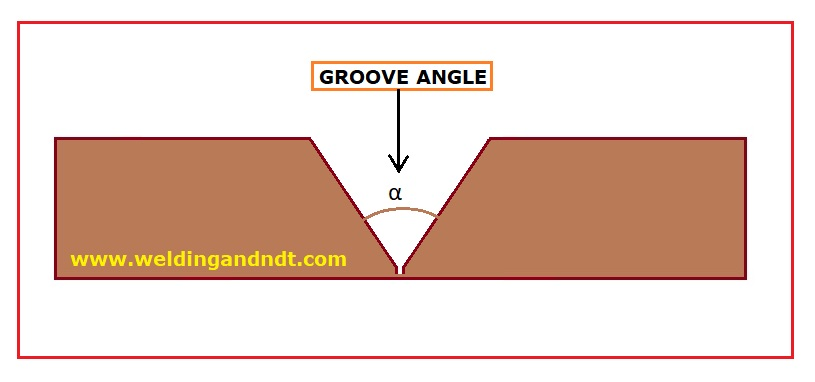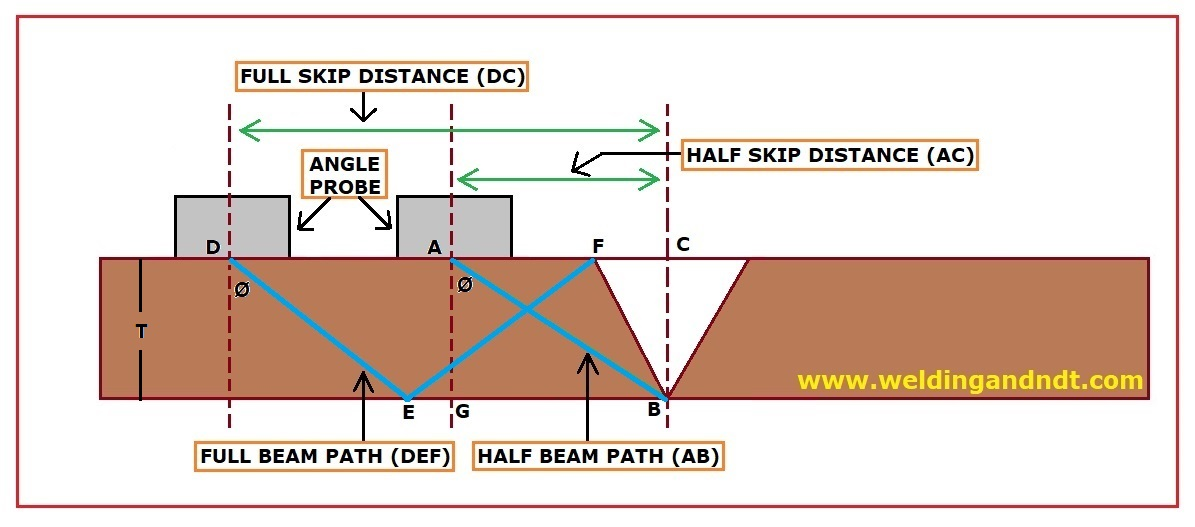# Angle Probe Calculation for UT

### SELECTION OF PROBE ANGLE:

There are three methods for selecting a probe angle, these are:

1. ##### Based on groove angle:

Probe Angle (Ø) = 90 – α/2

Where, α – Groove angle and Ø- Probe Angle1. ##### As per AWS:
• 0 – 30 mm Thickness – 700 Probe
• 30 – 40 mm Thickness – 600 Probe
• > 40 mm Thickness – 450 Probe
1. ##### As per approved procedure:

Third way of selection of Probe angle is as per the procedure approved by any competent person/client.

## SKIP DISTANCE AND BEAM PATH (SOUND PATH) CALCULATION:1. ### Skip distance calculation:

(Abbreviations: HSD – Half Skip Distance, FSD – Full Skip Distance)

Tan Ø = Perpendicular (BG)/ Base (AG) = AC (or HSD)/THK

Hence, Tan Ø = HSD/T

Or, HSD = T X Tan Ø

Similarly, FSD = 2T X Tan Ø

And, 1-1/2 Skip Distance = 3T X Tan Ø

1. ### Beam Path calculation:

(Abbreviations: HBP – Half Beam Path, FBP – Full Beam Path)

Cos Ø = Base (AG) / Hypotenuse (AB) = THK/HBP

Or, HBP = T / Cos Ø

Similarly, FBP = 2T / Cos Ø

And, 1-1/2 Beam Path = 3T / Cos Ø

NOTE: 1. Scanning area should be 1-1/2 Skip Distance i.e. 3T X Tan Ø

2. Probe Travel Speed shall not be more than 150 mm/S

Read more: Preheating – How, When and Why

Read more: Thickness range as per ASME Section IX#### Sandeep Anand

I am a Mechanical Engineer with more than ten years of work experience in the field of welding and NDT.

### 3 thoughts on “Angle Probe Calculation for UT”

•April 18, 2019 at 11:35 pm

•April 18, 2019 at 11:36 pm

•